## 金属玻璃基复合材料的变形行为及本构关系研究综述 1)

* 西南交通大学力学与工程学院,应用力学与结构安全四川省重点实验室,成都 610031

† 中国科学院力学研究所,北京 100190

## REVIEW ON THE DEFORMATION BEHAVIOR AND CONSTITUTIVE EQUATIONS OF METALLIC GLASS MATRIX COMPOSITES 1)

Zhang Juan*, Kang Guozheng,*,2), Rao Wei

* Applied Mechanics and Structure Safety Key Laboratory of Sichuan Province,School of Mechanics and Engineering,Southwest Jiaotong University,Chengdu 610031,China

† Institute of Mechanics, Chinese Academy of Sciences,Beijing 100190,China

 基金资助: 1)国家自然科学基金资助项目.  11372259国家自然科学基金资助项目.  11532010

Received: 2020-02-15   Accepted: 2020-03-6   Online: 2020-03-18Abstract

Metallic glass and metallic glass matrix composites have good application prospects because of their excellent mechanical properties, and now more and more researches have been conducting on them. The deformation behavior, toughening mechanism and constitutive relationship of metallic glass matrix composites are summarized and reviewed in this paper, based on the existing research results in literature by other groups and the latest work done by the authors. Firstly, the research progress in the deformation behavior, failure mechanism and constitutive relation of metallic glass in recent decades is briefly reviewed. Then, the state-of-the-arts in the deformation behavior and failure mechanism of metallic glass matrix composites are introduced from the aspects of experiments and numerical simulation, and the plastic deformation, toughening mechanism and their correspondent influencing factors of metallic glass matrix composites are summarized. Furthermore, the existing studies on the constitutive equations of metallic glass matrix composites are reviewed, with emphasis on the application of homogenization method in this field. In addition, a two-stepped homogenization method proposed by the authors is introduced in more details as a representative approach, and then the constitutive model established on the two-stepped homogenization method and with a help of a failure criterion obtained by introducing a concentration of nano-voids as an internal variable is addressed. The deformation and failure behavior of metallic glass matrix composites are predicted reasonably by the proposed constitutive model. Finally, the research progress of this field is briefly summarized, and some future topics are suggested.

Keywords： metallic glass matrix composites ; deformation behavior ; toughening mechanism ; failure mechanism ; constitutive equation

Zhang Juan, Kang Guozheng, Rao Wei. REVIEW ON THE DEFORMATION BEHAVIOR AND CONSTITUTIVE EQUATIONS OF METALLIC GLASS MATRIX COMPOSITES 1). Chinese Journal of Theoretical and Applied Mechanics[J], 2020, 52(2): 318-332 DOI:10.6052/0459-1879-20-038

## 1 金属玻璃的变形机理及本构关系

### 1.1 金属玻璃的变形行为与失效机理研究

Trexler和Thadhani总结认为,金属玻璃的宏观塑性屈服与失效过程由许多小尺度事件组成,包括：(1)原子重新排列以适应施加的剪切应变,引起剪切变形区的形核;(2) 剪切局部化传播或者剪切带生长;(3) 局部变形区域绝 热加热;(4)剪切带或剪切带附近纳米晶的成核;(5) 纳米孔洞在剪切带内的成核;(6) 孔洞聚结导致的破坏.已有的实验[28-30]给出了一些证明这些小尺度事件的微观图像,如Wright等观察到Pd$_{40}$Ni$_{40}$P$_{20}$轴向压缩试样的断裂面上有凝固的液滴(图3),表明在断裂过程中有温度升高导致的局部融化现象;Jiang等捕捉到Al$_{90}$Fe$_{5}$Gd$_{5}$在压缩区中剪切带内产生的纳米晶粒(图4);Jiang等则给出了Zr$_{41.2}$Ti$_{13.8}$Cu$_{10}$Ni$_{12.5}$Be$_{22.5}$压缩断面上由微孔洞形成的蜂窝状结构的图像(图5).由此可见,剪切带的形核与扩展是块体金属玻璃非弹性变形的主要机制,而剪切带内剧烈的局部剪切将会诱导微裂纹在剪切带内形核,进而导致块体金属玻璃发生宏观脆性失效.

### 图1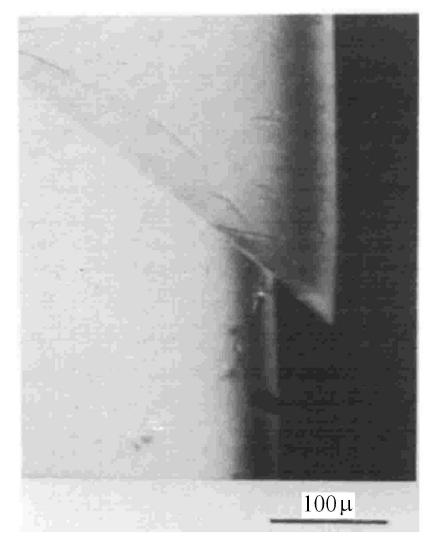Fig. 1   Localized shear deformation bands of Pd$_{0.775}$Cu$_{0.06}$Si$_{0.16}$

### 图2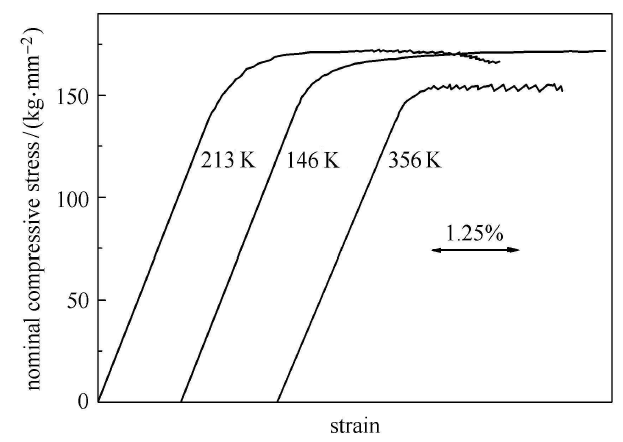Fig. 2   Compression stress-strain curves of Pd$_{0.775}$Cu$_{0.06}$Si$_{0.16}$ at different temperatures

### 图3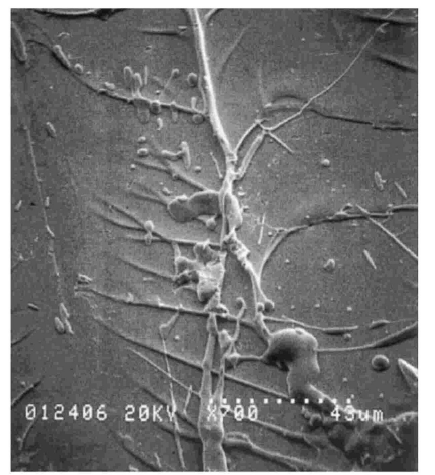Fig. 3   Scanning electron micrograph of the fracture surface of Pd$_{40}$Ni$_{40}$P$_{20}$ sample failed in uniaxial compression 

### 图4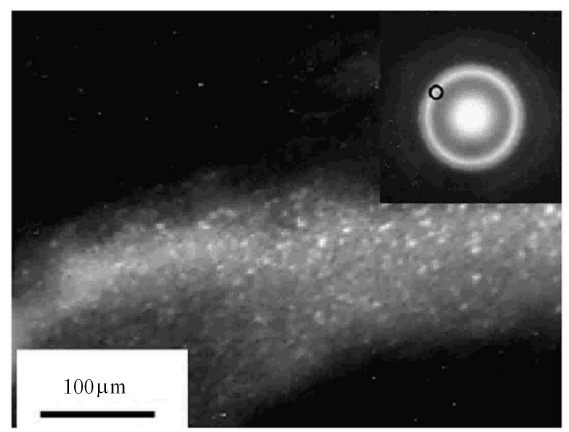Fig. 4   TEM image of the compressive region of Al$_{90}$Fe$_{5}$Gd$_{5}$, showing a number of nano-crystallites at a shear band

### 图5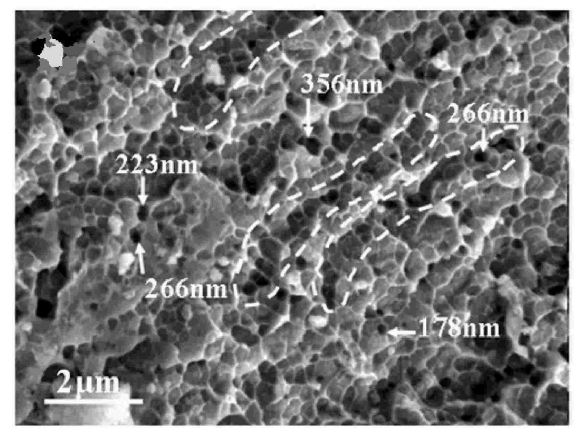Fig. 5   Honeycomb structures of the compressive fracture surface of a Zr$_{41.2}$Ti$_{13.8}$Cu$_{10}$Ni$_{12.5}$Be$_{22.5}$ sample 

### 1.2 金属玻璃的本构关系研究

(1) "自由体积"模型

1977年,Spaepen通过发展描述玻璃和液体扩散流动的经典"自由体积"理论,建立了金属玻璃塑性流动的自由体积模型.Spaepen认为,在应力驱动下,单个原子以类似扩散跳跃的方式导致自由体积的产生,一系列离散的原子跳跃最终引起宏观上的塑性流动;进而以此为基础,建立了以自由体积为内变量的金属玻璃塑性流动本构关系,其中,宏观塑性剪切应变率$\dot {\gamma }$的表达式如下

$\dot {\gamma } = \Delta f\exp \left( { - \dfrac{\chi \upsilon ^\ast }{\upsilon _{\rm f} }} \right)2\nu \sinh \left( {\dfrac{\tau \varOmega }{2k_{\rm B} T}} \right)\exp \left( { - \dfrac{\Delta G^{\rm m}}{k_{\rm B} T}} \right)$

$\dot {\upsilon }_{\rm f} = \upsilon ^\ast \nu \exp \left( { - \dfrac{\chi \upsilon ^\ast }{\upsilon _{\rm f} }} \right)\exp \left( { - \dfrac{\varDelta G^{\rm m}}{k_{\rm B} T}} \right)\cdot \\ \left\{ {\dfrac{2\chi k_{\rm B} T}{\upsilon _{\rm f} S}\left[ {\cosh \left( {\dfrac{\tau \Omega }{2k_{\rm B} T}} \right) - 1} \right] - \dfrac{1}{n_{\rm D} }} \right\}$

$\omega _{\rm cr} = \omega _t + {\rm sign}\left( \phi \right){\rm e}^{ - \left| \phi \right|}\omega _{\rm sh}$

### 图6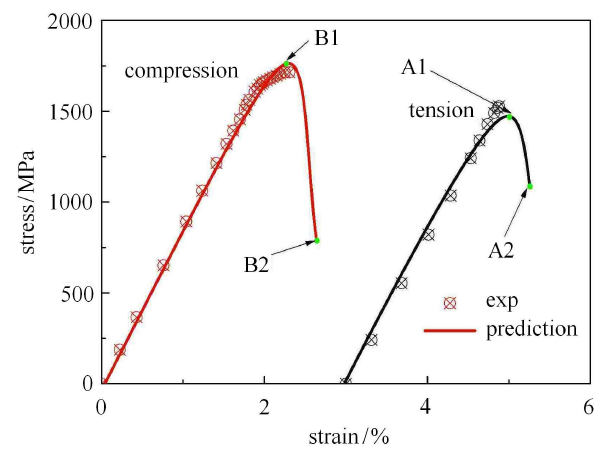Fig. 6   Comparison between the simulated stress-strain curves and experimental ones 

### 图7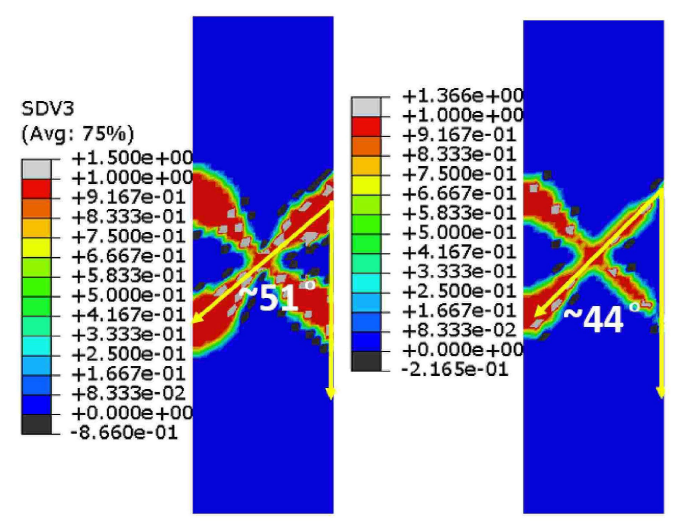Fig. 7   Prediction of tension and compression fracture angle 

(2) "剪切转变"模型

1979年,Argon在自由体积模型的基础上提出了剪切转换模型. 与自由体积模型相比,剪切转换模型认为金属玻璃塑性变形的最基本方式是原子团簇的协同重排,而非是单个原子的跃迁.在热-力耦合作用下,材料通过一系列的"剪切转变"过程,容纳局部的塑性剪切变形,最终以一种类似雪崩自组织的方式导致宏观尺度的塑性流动.Argon进一步认为,这些"剪切转变"过程并不是自由的,而是被周围没有发生"剪切转变"的弹性介质所约束.因此,"剪切转变"类似于经典的Eshelby夹杂问题,将在周围弹性介质中产生各向异性的弹性应力场.基于Eshelby的等效夹杂理论,Argon计算了这种"剪切转变''的激活自由能,如下式所示

$\Delta F_0 = \left[ {\dfrac{7 - 5\nu _0 }{30\left( {1 - \nu _0 } \right)} + \dfrac{1\left( {1 + \nu _0 } \right)}{9\left( {1 - \nu _0 } \right)}l^2 + \dfrac{1}{2\gamma _0 }\dfrac{\tau _0 }{\mu \left( T \right)}} \right]\mu \left( T \right)\gamma _0^2 \varOmega _0$

$\dot {\gamma } = \alpha _0 \nu \gamma _0 \exp \left( { - \dfrac{\varDelta F}{k_{\rm B} T}} \right)\sinh \left( {\dfrac{\tau V_{\rm a} }{k_{\rm B} T}} \right)$

$\alpha _0 \propto \exp \left( { - \dfrac{\chi \upsilon ^\ast }{\upsilon _{\rm f} }} \right)$

"剪切转变"理论提出以后,学者们对此进行了一系列的实验和模拟研究,但是,直到2007 年Schall等才通过一 种胶体玻璃的剪切实验,获得了这种"剪切转变"的实验图像.目前,剪切转换模型已经得到广泛的认可.近年来,为了解释块体金属玻璃的韧脆转换,Jiang等通过一系列实验探究了不同加载条件下块体金属玻璃的变形与失效行为,并在剪切转换模型的基础上提出了拉伸转换模型.拉伸转换模型是剪切转换模型的一种很好的补充,两种模型联合起来能够解释很多块体金属玻璃失效相关的实验现象,例如断裂模式的演化、拉压非对称及韧-脆转换等.

(3) 其他模型

## 2 金属玻璃基复合材料的变形与失效行为

### 2.1 实验研究

20世纪90年代以来,国内外学者对各种不同制备方式得到的块体金属玻璃基复合材料的力学性能进行了一系列的实验研究,取得了较多研究成果.实验结果表明,不管是内生法还是外加法,第二相的加入都不同程度地改善了块状金属玻璃的塑性变形能力.例如：Johnson研究小组通过外加法制备了含WC,SiC,W或Ta颗粒的大块金属玻璃基复合材料,其压缩塑性应变为5%;Chen等通过内生晶相法制备的Zr基金属玻璃复合材料,其拉伸塑性应变可达6.2%.目前报道的块体金属玻璃基复合材料室温下单调拉伸时的最大塑性应变可达15%以上,如图8所示;而压缩时的最大塑性应变可达50%,如图9所示. 另外,已有的实验研究表明[59-60],金属玻璃基复合材料的单调拉伸和压缩力学性能不相同;同时,其拉伸和压缩力学性能对温度和加载率都很敏感.例如,Li等发现外加Ta颗粒的Zr基金属玻璃复合材料在过冷液相区的单调压缩力学性能具有明显的温度依赖性和加载率依赖性.

### 图8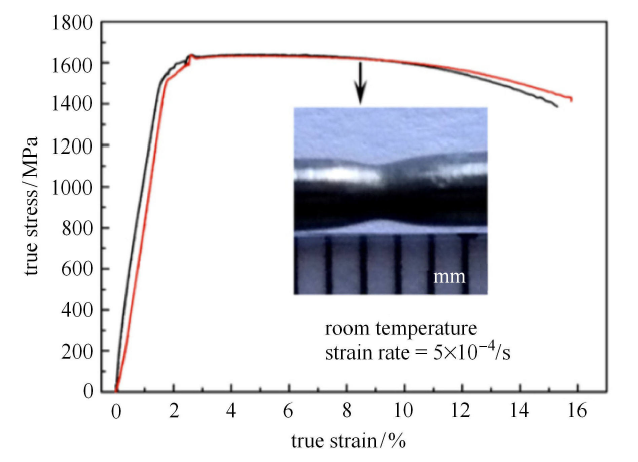Fig. 8   Tensile true stress-strain curves of Ti$_{46}$Zr$_{20}$V$_{12}$Cu$_{5}$Be$_{17}$ at room temperature 

### 图9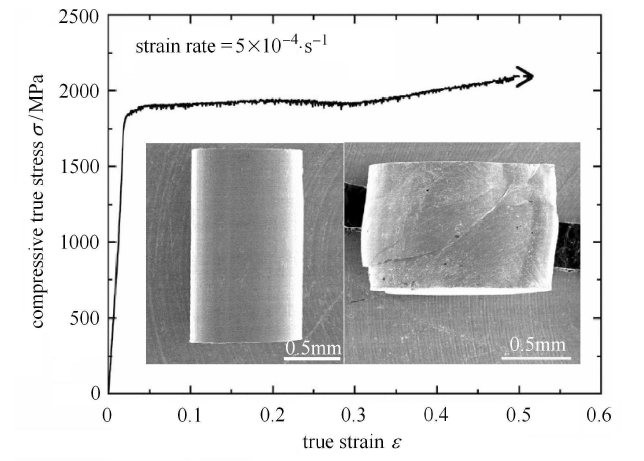Fig. 9   True stress-true strain curve for Cu$_{50}$Zr$_{50}$ loaded in uniaxial compression 

Table 1  Compressive mechanical properties formetallic glass matrix composites with different Ta contents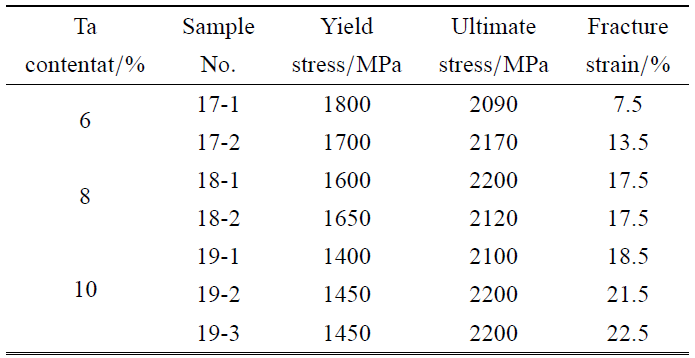### 图10Fig. 10   Tensile engineering stress-strain curves for the metallic glass matrix composites with different size $\beta$-Zr particles

### 2.2 数值模拟

Zhou等首先对金属玻璃基复合材料的变形行为进行了分子动力学模拟,模拟结果表明：晶体第二相在金属玻璃基体中引入了额外的结构长度尺度.通过将这种结构长度尺度与金属玻璃基体的固有长度尺度(例如新形成的剪切带的厚度)相匹配,可以使剪切转变区均匀形核或多剪切带萌生,从而提高复合材料整体塑性.图11给出了Zhou等模拟的剪切带与晶体第二相之间的交互作用图像.Zhou等的研究结果还表明,晶体相(即增韧相)与基体之间的界面对于复合材料的塑性变形和失效具有重要的作用,具体体现为：在变形过程中首先是金属玻璃中的塑性剪切被纳米晶体阻挡,局部剪切带被迫沿玻璃与晶体界面偏转,然后纳米晶才出现晶格滑移;此后,位错在玻璃与晶体界面上形核,同时在附近的非晶基体上形成剪切转变区,以容纳与晶格位错相关的剪切位移.当施加的应变增加时,大多数位错将被纳米晶对面的玻璃与晶体界面吸收;同时,许多剪切转变区在玻璃与晶体界面的吸收点位附近被激活,以充分容纳由位错造成的局部剪切应变.

### 图11Fig. 11   Typical atomistic configurations illustrating the interaction between the operating shear bands and the second-phase nanocrystals

Jiang等[84-85]利用有限元方法,在代表性体积单元的基础上对块体金属玻璃基复合材料的单调拉伸变形行为进行了数值模拟,探讨了单调拉伸过程中剪切带的演化规律以及增韧相的体积分数、力学性能及形貌对块体金属玻璃基体中剪切带扩展的影响.图12给出了Jiang等模拟的金属玻璃基复合材料在单轴拉伸载荷下局部剪切带的演化过程.

### 图12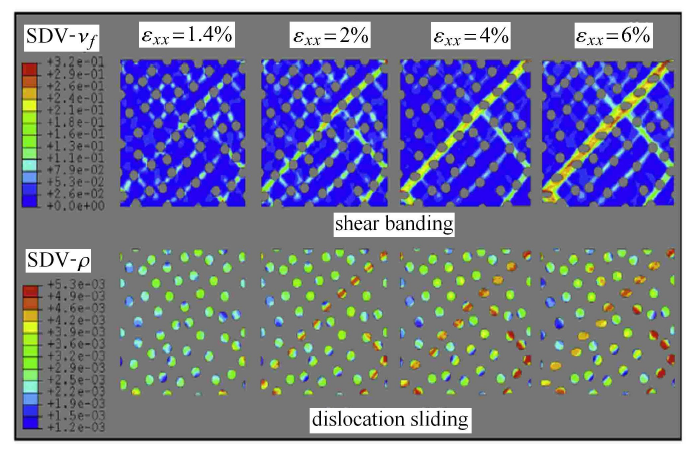Fig. 12   Evolutions of dislocation sliding in ductile particles and shear banding in bulk metallic glass matrix with the applied macroscopic deformation for bulk metallic glass matrix composites 

Shete等[86-87]则利用有限元方法探究了增韧相的应变硬化、体积分数和形貌对基体内剪切带形核与扩展的影响.由于Shete等[86-87]采用了大变形非局部本构关系和晶体塑性模型分别来描述块体金属玻璃基体及增韧相的变形行为,因此模拟结果很好地捕获了剪切带的宽度、增韧相的塑性剪切与晶格旋转等微观结构的演化.另外,已有学者[88-92]利用有限元方法研究了单调拉伸变形过程中增韧相的大小、几何结构、相变、孪生及界面强度等因素对块体金属玻璃基复合材料宏观变形行为及微观结构演化的影响.这些工作的关注点各不相同,例如：Chu等讨论了界面强度对锆基块体金属玻璃基复合材料变形行为的影响;Jeon等研究了枝晶相的尺寸对块体金属玻璃基复合材料变形行为的影响.

### 图13Fig. 13   Shear bands of the bulk metallic glass matrix composites with different particle volume fractions in tension ((a), (c) and (e)) and in compression ((b), (d) and (f)) at the strain $\varepsilon _{xx}=5\%$

## 3 金属玻璃基复合材料的本构关系

### 3.1 基于均匀化方法的本构关系

$\dot {\gamma }_{\rm (M)} = sign\left( {\bar {\tau }_{\rm (M)} } \right)\dot {\gamma }_{\rm 0(M)}\left(\frac{| {\bar {\tau }_{\rm (M)} } |}{s_{\rm (M)} + \mu {\bar{P}_{\rm (M)} }} \right)^{1 /{n_{\rm (M)} }}$

$\dot {s}_{\rm (M)} = h_{0\rm (M)} \left[ {s_{k\rm (M)} \left( {1 - \beta _d \exp ( - \dfrac{\varphi }{\xi })} \right) - s_{\rm (M)} } \right]\dot {\gamma }_{\rm (M)}$

$\dot {\xi } = \varsigma \dot {\gamma }_{\rm (M)} - \left( {\dfrac{\dot {\gamma }_{0\rm (M)} }{s_{\xi _{II} } }} \right)\bar {P}_{\rm (M)} - \left( {\dfrac{\dot {\gamma }_{0\rm (M)} s_{\xi _I } }{s_{\xi _{II} } }} \right)\left( {\xi - \xi _T } \right)$

$\dot {\gamma }_{\rm (I)} = {\rm sign}\left( {\bar {\tau }_{\rm (I)} } \right)\dot {\gamma }_{0\rm (I)} \left( {\dfrac{\left| {\bar {\tau }_{\rm (I)} } \right|}{s_{\rm (I)} }} \right)^{1/{n_{\rm (I)} }}$

$\dot {s}_{\rm (I)} = h_{0\rm (I)} \left\langle {1 - \dfrac{s_{\rm (I)} }{s_{c\rm (I)}^\ast }} \right\rangle ^{ a}\dot {\gamma }_{\rm (I)}$

$\left.\begin{array}{l@{\quad}l} {\pmb F } = {\pmb F }_{\rm (M)} = {\pmb F }_{\rm (I)} \\ {\pmb T} = \left( {1 - v_{\rm I} } \right) {\pmb T }_{\rm (M)} + v_{\rm I} {\pmb T }_{\rm (I)} \end{array}\right\}$

Jiang等将Weng等提出的韧性材料的细观本构模型进行了拓展,构建了一个能够描述块体金属玻璃基复合材料变形行为的细观本构模型. 该模型中,采用自由体积模型描述基体相的变形;而夹杂相的演化方程为

$\left.\begin{array}{l@{\quad}l} \sigma _{\rm eq} = \sigma _{y\rm (I)} + h\left( {\varepsilon _{\rm eq(I)}^{\rm p} } \right)^n \\ \varepsilon _{ij\rm (I)}^{\rm p} = \dfrac{3}{2}\dfrac{\varepsilon _{\rm eq(I)}^{\rm p} }{\sigma _{\rm eq(I)} }{\sigma }'_{ij\rm (I)} \end{array}\right\}$

$\left.\!\!\begin{array}{l@{\quad}l}\bar {\sigma }_{kk} = 3 \kappa _{\rm (M)} \left[ {1 + \dfrac{v_{\rm (I)} \left( {\kappa _{\rm (I)} - \kappa _{\rm (M)} } \right)}{v_{\rm (M)} \alpha _{\rm (M)}^s \left( {\kappa _{\rm (I)} - \kappa _{\rm (M)} } \right) + \kappa _{\rm (M)} }} \right]\bar {\varepsilon }_{kk} \\ {\sigma }'_{ij} = 2\mu _{\rm (M)}^{\rm s} \left[ {1 + \dfrac{{\rm c}_{\rm (I)} \left( {\mu _{(I)} - \mu _{\rm (M)} } \right)}{c_{\rm (M)} \beta _{\rm (M)}^s \left( {\mu _{\rm (I)} - \mu _{\rm (M)} } \right) + \mu _{\rm (M)} }} \right]{\bar {\varepsilon }}'_{ij} -\\ \qquad \dfrac{{\rm c}_{\rm (I)} \mu _{\rm (I)} }{c_{\rm (M)} \beta _{\rm (M)}^s \left( {\mu _{\rm (I)} - \mu _{\rm (M)} } \right) + \mu _{\rm (M)} }\bar {\varepsilon }_{ij\rm (I)}^{ p} \end{array}\!\!\right\}$

$\left.\!\!\begin{array}{l@{\quad}l}\dfrac{E}{E_{{\rm in}} } = \left[ {1 + \dfrac{16\left( {1 - \phi _{\rm in}^2 } \right)\left( {1 - {3\phi _{\rm in} }/ {10}} \right)}{9\left( {1 - {\phi _{\rm in} }/2} \right)}\rho } \right]^{ - 1} \\ \dfrac{\mu }{\mu _{\rm in} } = \left[ 1 + \dfrac{16\left( {1 - \phi _{\rm in} } \right)\left( {1 -\phi _{\rm in}/ 5} \right)}{9\left( 1 - \phi _{\rm in} /2 \right)} \rho \right]^{ - 1} \\ \dfrac{\varphi }{\phi _{\rm in} } = \dfrac{E}{E_{\rm in} }\left[ {1 + \dfrac{8\left( {1 - \phi _{\rm in}^2 } \right)}{45\left( {1 - {\phi _{\rm in} } / 2} \right)}\rho } \right] \end{array}\!\!\right\}$

$\rho = \rho _0 \left\{ {1 - \exp \left[ {1 - \left( {\dfrac{\varepsilon ^P}{\varepsilon _0 }} \right)^m} \right]} \right\}\left( {1 - v_I } \right)\left[ {1 - \left\langle {v_{\rm cr} - v_I } \right\rangle ^\chi } \right]$

### 图14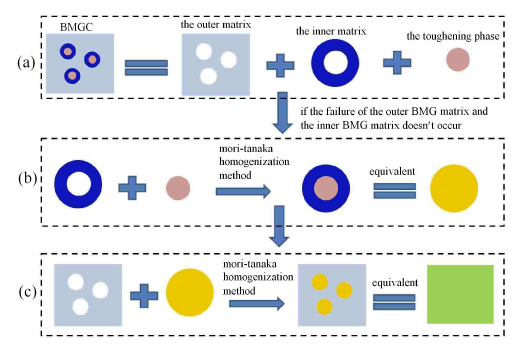Fig. 14   Diagram for the two-stepped homogenization method: (a) the relation between the bulk metallic glass matrix composites and three phases in the composites; (b) the first homogenization for the inner matrix and TP; (c) the second homogenization for the outer matrix and equivalent inclusion 

### 图15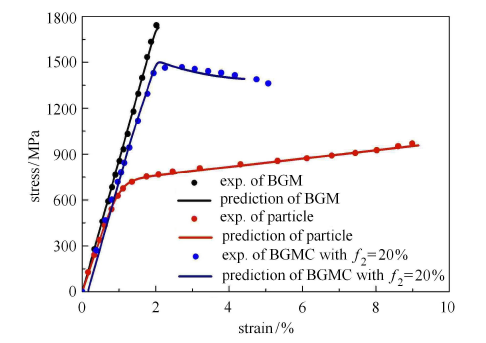Fig. 15   Modeled and experimental stress-strain responses of Zr-based bulk metallic glass matrix composites 

### 图16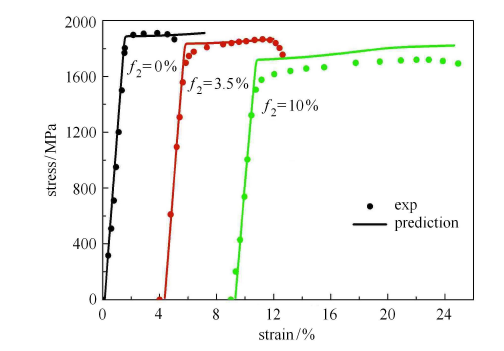Fig. 16   Modeled and experimental stress-strain responses of bulk metallic glass matrix composites containing graphite TP 

## 4 结语

(1) 采用多尺度分析方法(包括实验和数值模拟),进一步理清金属玻璃基体以及金属玻璃基复合材料塑性变形机制和增韧机制的物理图像.

(2) 发展能够全面考虑微结构参数,如体积分数、增韧相尺寸、界面等影响的金属玻璃基复合材料本构关系.

(3) 发展能够合理反映金属玻璃基复合材料增韧机理,并能准确预测复合材料失效行为的本构关系.

(4) 进行循环加载和非比例多轴加载等复杂载荷作用下金属玻璃基复合材料力学行为的研究,包括实验研究、数值模拟和本构关系构建,为该类材料的发展和工程应用提供坚实的理论支持.

## 参考文献 原文顺序 文献年度倒序 文中引用次数倒序 被引期刊影响因子

Klement W, Willens RH, Duwez P .

Non-crystalline structure in solidified gold-silicon alloys

Nature, 1960,187:869-870

Inoue A, Zhang T, Masumoto T .

Al-La-Ni Amorphous alloys with a wide supercooled liquid region. Mater Trans,

JIM, 1989,30:965-972

Peker A, Johnson WL .

A highly processable metallic glass: Zr$_{41.2}$Ti$_{13.8}$Cu$_{12.5}$Ni$_{10.0}$Be$_{22.5}$

Appl Phys Lett, 1993,63:2342-2344

( Hu Zhuangqi, Zhang Haifeng .

Recent progress in the area of bulk armorphous alloys and composites

Acta Metallurgica Sinica, 2010,46(11):1391-1421 (in Chinese))

Choi-yim H, Busch R, Koester U , et al.

Synthesis and characterization of particulate reinforced Zr$_{57}$Nb$_{5}$Al$_{10 }$Cu$_{15.4}$Ni$_{12.6}$ bulk metallic composites

Acta Mater, 1999,47:2455-2462

Chen G, Cheng JL, Liu CT .

Large-sized Zr-based bulk- metallic-glass composite with enhanced tensile properties

Intermetallics, 2012,28:25-33

Qiao JW, Sun AC, Huang EW , et al.

Tensile deformation micro-mechanisms for bulk metallic glass matrix composites: From work-hardening to softening

Acta Mater., 2011,59:4126-4137

Qiao JW, Jia H, Liaw PK .

Metallic glass matrix composites

Mater Sci Eng R, 2016,100:1-69

Kato H, Inoue A .

Synthesis and mechanical properties of bulk amorphous Zr-Al-Ni-Cu alloys containing ZrC particles.

Mater, Trans, 1997,38:793-800

Ma G, Zhang HF, Li H , et al.

Wetting behavior of CuZr-based BMGs/alumina system

J Alloys and Compounds, 2008,462:343-346

Liu N, Ma G, Zhang HF , et al.

Wetting behavior of Zr-based bulk metallic glasses on W substrate

Mater Lett, 2008,62:3195-3197

Li JB, Jang JSC, Li C , et al.

Significant plasticity enhancement of Zr Cu-based bulk metallic glass composite dispersed by in situ and ex situ Ta particles

Mater Sci Eng A, 2012,551:249-254

Mechanical properties of bulk metallic glasses

Progr Mater Sci, 2010,55:759-839

Wang WH .

The elastic properties, elastic models and elastic perspectives of metallic glasses

Progress in Materials Science, 2012,57:487-656

Dai LH.

Shear Banding in Bulk Metallic Glasses

In: Dodd B, Bai YL, eds. Adiabatic Shear Localization: Frontiers and Advances. Massachusetts: Elsevie, 2012. 311-361

( Jiang Minqing .

Advances in plasticity theory for amorphous alloys

Materials China, 2014,33(5):257-264(in Chinese))

( Lei Xianqi, Wei Yujie .

The strength and deformation behavior of metallic glasses

Chinese Journal of Solid Mechanics, 2016,37(4):312-339 (in Chinese))

Volkert CA, Donohue A, Spaepen F .

Effect of sample size on deformation in amorphous metals

J Appl Phys, 2008,103:083539

Wu F, Zhang Z, Mao SX .

Size-dependent shear fracture and global tensile plasticity of metallic glasses

Acta Mater, 2009,57:257-266

Jang D, Greer JR .

Transition from a strong-yet-brittle to a stronger-and-ductile state by size reduction of metallic glasses

Nature Mater, 2010,9:215-219

Chen D, Jang D, Guan KM , et al.

Nanometallic glasses: size reduction brings ductility, surface state drives its extent

Nano Lett, 2013,13:4462-4468

Polk DE, Turnbull D .

Flow of melt and glass forms of metallic alloys

Acta Metall, 1972,20:493-498

Pampillo CA .

Localized shear deformation in a glassy metal

Scripta Metall, 1972,6:915-917

Chen HS, Leamy HJ, Obrien MJ .

Bending deformation in metallic glasses

Scripta Metall, 1973,7:415-419

Greer AL, Cheng YQ, Ma E .

Shear bands in metallic glasses

Mater Sci Eng R, 2013,74(4):71-132

Schuster BE, Wei Q, Ervin MH , et al.

Bulk and microscale compressive properties of a Pd-based metallic glass

Scripta Mater, 2007,57:517-520

Pampillo CA, Chen HS .

Comprehensive plastic deformation of a bulk metallic glass

Mater Sci Eng A, 1974,13:181-188

Wright WJ, Schwarz RB, Nix WD .

Localized heating during serrated plastic flow in bulk metallic glasses

Mater Sci Eng A, 2001, 319-321:229-232

Jiang WH, Atzmon M .

The effect of compression and tension on shear-band structure and nanocrystallization in amorphous Al90Fe5Gd5: A high-resolution transmission electron microscopy study

Acta Mater, 2003,51:4095-4105

Jiang MQ, Ling Z, Meng JX , et al.

Energy dissipation in fracture of bulk metallic glasses via inherent competition between local softening and quasi-cleavage

Phil Mag, 2008,88:407-426

Sun BA, Wang WH .

The fracture of bulk metallic glasses

Prog Mater Sci, 2015,74:211-307

Spaepen F .

A microscopic mechanism for steady state inhomogeneous flow in metallic glasses

Acta Metall, 1977,25:407-415

Johnson WL, Lu J, Demetriou MD .

Deformation and flow in bulk metallic glasses and deeply undercooled glass forming liquids-A selfconsistent dynamic free volume model

Intermetallics, 2002,10:1039-1046

Anand L, Su C .

A theory for amorphous viscoplastic materials undergoing finite deformations, with application to metallic glasses

J Mech Phys Solids, 2005,53:1362-1396

Yang Q, Mota A, Ortiz M .

A Finite-deformation constitutive model of bulk metallic glass plasticity

Comput Mech, 2006,37:194-204

Gao YF, Yang B, Nieh TG .

Thermomechanical instability analysis of inhomogeneous deformation in amorphous alloys

Acta Mater, 2007,55:2319-2327

Thamburaja P, Ekambaram R .

Coupled thermo-mechanical modelling of bulk-metallic glasses: Theory, finite-element simulations and experimental verification

J Mech Phys Solids, 2007,55:1236-1273

Huang R, Suo Z, Prevost JH , et al.

Inhomogeneous deformation in metallic glasses

J Mech Phys Solids, 2002,50:1011-1127

Jiang MQ, Dai LH .

On the origin of shear banding instability in metallic glasses

J Mech Phys Solids, 2009,57:1267-1292

Thamburaja P .

Length scale effects on the shear localization process in metallic glasses: A theoretical and computational study

J Mech Phys Solids, 2011,59:1552-1575

Rao W, Zhang J, Kang GZ .

A failure mechanism based constitutive model for bulk metallic glass

Mech Mater, 2018,125:52-69

Argon AS .

Plastic deformation in metallic glasses

Acta Metall, 1979,27:47-58

Eshelby JD .

The Determination of the elastic field of an ellipsoidal inclusion, and related problems

Proc Roy Soc London A, 1957,241:376-396

Spaepen F. Defects in amorphous metals//Balian R, Kleman M, Poirier J. Physics of Defects. Amsterdam: North-Hollan Press, 1981: 133-174

Schall P, Weitz DA, Spaepen F .

Structural rearrangements that govern flow in colloidal glasses

Science, 2007,318:1895-1899

Jiang MQ, Ling Z, Meng JX , et al.

Energy dissipation in fracture of bulk metallic glasses via inherent competition between local softening and quasi-cleavage

Phil Mag, 2008,88:407-426

Falk ML, Langer JS .

Dynamics of viscoplastic deformation in amorphous solids

Phys Rev E, 1998,57:7192-7205

Malandro DL, Lacks DJ .

Relationships of shear-induced changes in the potential energy landscape to the mechanical properties of ductile glasses

J Chem Phys, 1999,110:4593-4601

Langer JS .

Dynamics of shear-transformation zones in amorphous plasticity: Formulation in terms of an effective disorder temperature

Phys Rev E, 2004,70:041502

Demetriou MD, Harmon JS, Tao M , et al.

Cooperative shear model for the rheology of glass-forming metallic liquids

Phys Rev Lett , 2006,97:065502

Jiao W, Sun BA, Wen P , et al.

Crossover from stochastic activation to cooperative motions of shear transformation zones in metallic glasses

Appl Phys Lett, 2013,103:081904

Zhu Z, Wen P, Wang DP , et al.

Characterization of flow units in metallic glass through structural relaxations

J Appl Phys, 2013,114:083512

( Wang Zheng, Wang Weihua .

Flow unit model in metallic glasses

Acta Phys Sin, 2017,66(17):176103 (in Chinese))

( Wang Weihua .

Flow units: the "defects" of amorphous alloys

Scientia Sinica: Physica, Mechanica & Astronomica, 2014,44(4):396-405 (in Chinese))

Choi-yim H, Busch R, Koester U , et al.

Synthesis and characterization of particulate reinforced Zr$_{57}$Nb$_{5}$Al$_{10 }$Cu$_{15.4}$Ni$_{12.6}$ bulk metallic composites

Acta Mater, 1999,47:2455-2462

Chen G, Cheng JL, Liu CT .

Large-sized Zr-based bulk-metallic-glass composite with enhanced tensile properties

Intermetallics, 2012,28:25-33

Qiao JW, Sun AC, Huang EW , et al.

Tensile deformation micromechanisms for bulk metallic glass matrix composites: From work-hardening to softening

Acta Mater, 2011,59:4126-4137

Inoue A, Zhang W, Tsurui T , et al.

Unusual room- temperature compressive plasticity in nanocrystal-toughened bulk copper-zirconium glass

Phil Mag Lett, 2005,85:221-229

Szuecs F, Kim CP, Johnson WL .

Mechanical properties of Zr$_{56.2}$Ti$_{13.8}$Nb$_{5.0}$Cu$_{6.9}$Ni$_{5.6}$Be$_{12.5}$ ductile phase reinforced bulk metallic glasses composite

Acta Mater, 2001,49:1507-1513

Li JB, Zhang HZ, Jang JSC , et al.

Viscous flow and thermoplastic forming ability of a Zr-based bulk metallic glass composite with Ta dispersoids

J Alloys and Compounds, 2012,536S:S165-S170

Conner RD, Choi-Yim H, Johnson WL .

Mechanical properties of Zr$_{57}$Nb$_{5}$Al$_{10}$Cu$_{15.4}$Ni$_{12.6}$ metallic glass matrix particulate composites

J Mater Res, 1999,14:3292-3297

Qiu KQ, Wang AM, Zhang HF , et al.

Mechanical properties of tungsten fiber reinforced ZrAlNiCuSi metallic glass matrix composite

Intermetallics, 2002,10:1283-1288

Dong W, Zhang H, Sun WS , et al.

Zr-Cu-Ni-Al-Ta glassy matrix composites with enhanced plasticity

J Mater Res, 2006,21:1490-1499

Siegrist ME, Löffler JF .

Bulk metallic glass-graphite composites

Scripta Mater, 2007,56:1079-1082

Jang JSC, Li TH, Tsai PH , et al.

Critical obstacle size to deflect shear banding in Zr-based bulk metallic glass composites

Intermetallics, 2015,64:102-105

Lee JC, Kim YC, Ahn JP , et al.

Enhanced plasticity in a bulk amorphous matrix composite: Macroscopic and microscopic viewpoint studies

Acta Mater, 2005,53:129-139

Hofmann DC, Suh JY, Wiest A , et al.

Designing metallic glass matrix composites with high toughness and tensile ductility

Nature, 2008,451:1085-1089

Jang JSC, Jian SR, Li TH , et al.

Structural and mechanical characterizations of ductile Fe particles-reinforced Mg-based bulk metallic glass composites

J Alloys and Compounds, 2009,485:290-294

Jang JSC, Ciou JY, Li TH , et al.

Dispersion toughening of Mg-based bulk metallic glass reinforced with porous Mo particles

Intermetallics, 2010,18:451-458

Pauly S, Gorantla S, Wang G , et al.

Transformation-mediated ductility in CuZr-based bulk metallic glasses

Nature Mater, 2010,9:473-477

Song K, Pauly S, Sun BA , et al.

Correlation between the microstructures and the deformation mechanisms of CuZr-based bulk metallic glass composites

Brink T, Peterlechner M, Rösner H , et al.

Influence of crystalline nanoprecipitates on shear-band propagation in Cu-Zr-based metallic glasses

Phys Rev Appl, 2016,5:054005

Sarac B, Schroers J .

Designing tensile ductility in metallic glasses

Nature Comm, 2013,4:2158

Lee SW, Huh MY, Fleury E , et al.

Crystallization-induced plasticity of Cu-Zr containing bulk amorphous alloys

Acta Mater, 2006,54:349-355

Song G, Lee C, Hong SH , et al.

Martensitic transformation in a B2-containing CuZr-based BMG composite revealed by in situ neutron diffraction

J Alloys and Compounds, 2017,72:714-721

Liu Y, Yao H, Zhang T , et al.

Designing ductile CuZr-based metallic glass matrix composites

Mater Sci Eng A, 2017,682:542-549

Zhou H, Qu S, Yang W .

An atomistic investigation of structural evolution in metallic glass matrix composites

Int J Plast, 2013,44:147-160

Avchaciov K, Ritter Y, Djurabekova F , et al.

Controlled softening of Cu64Zr36 metallic glass by ion irradiation

Appl Phys Lett, 2013,102:181910

Sopu D, Stoica M, Eckert J .

Deformation behavior of metallic glass composites reinforced with shape memory nanowires studied via molecular dynamics simulations

Appl Phys Lett, 2015,106:211902

Brandl C, Germann TC, Misra A .

Structure and shear deformation of metallic crystalline-amorphous interfaces

Acta Mater, 2013,61:3600-3611

Gao X, Muser MH, Kong LT , et al.

Atomic structure and energetics of amorphous-crystalline CuZr interfaces: A molecular dynamics study

Modell Simul Mater Sci Eng, 2014,22:065007

Shi Y, Falk ML .

A computational analysis of the deformation mechanisms of a nanocrystal-metallic glass composite

Acta Mater, 2008,56:995-1000

Cheng B, Trelewicz JR .

Mechanistic coupling of dislocation and shear transformation zone plasticity in crystalline-amorphous nanolaminates

Acta Mater, 2016,117:293-305

Jiang Y, Qiu K .

Computational micromechanics analysis of toughening mechanisms of particle-reinforced bulk metallic glass composites

Mater Des, 2015,65:410-416

Jiang Y, Shi X, Qiu K .

Numerical study of shear banding evolution in bulk metallic glass composites

Mater Des, 2015,77:32-40

Shete MK, Singh I, Narasimhan R , et al.

Effect of strain hardening and volume fraction of crystalline phase on strength and ductility of bulk metallic glass composites

Scripta Mater, 2016,124:51-55

Shete MK, Dutta T, Singh I , et al.

Tensile stress-strain response of metallic glass matrix composites reinforced with crystalline dendrites: Role of dendrite morphology

Intermetallics, 2017,83:70-82

Jiang Y, Sun L, Wu Q , et al.

Enhanced tensile ductility of metallic glass matrix composites with novel microstructure.

J Non-crystalline Solids, 2017,459:26-31

Fan J, Qiao JW, Wang Z , et al.

Twinning-induced plasticity (TWIP) and work hardening in Ti-based metallic glass matrix composites

Sci Rep, 2017,7:1877

Zhang X, Ren J, Ding X .

Synergistic effects among the structure, martensite transformation and shear band in a shape memory alloy-metallic glass composite

Appl Comp Mater, 2019,26:455-467

Chu Z, Yuan G, Kato H , et al.

The study on interface and property of TiNb/Zr-based metallic glassy composite fabricated by SPS.

J Non-crystalline Solids, 2015,426:83-87

Jeon C, Lee H, Kim CP , et al.

Effects of effective dendrite size on tensile deformation behavior in Ti-based dendrite-containing amorphous matrix composites modified from Ti-6Al-4V alloy

Metall Mater Trans A, 2015,46:235-250

Rao W, Zhang J, Kang GZ , et al.

Numerical simulation on the deformation behaviors of bulk metallic glass composites under uniaxial tension and compression

Comp Struct, 2018,187:411-428

Marandi K, Shim VPW .

A finite-deformation constitutive model for bulk metallic glass composites

Contin Mech Therm, 2014,26:321-341

Jiang Y .

Micromechanics constitutive model for predicting the stress-strain relations of particle toughened bulk metallic glass matrix composites

Intermetallics, 2017,90:147-151.

Weng GJ .

The overall elastoplastic stress-strain relations of dual-phase metals

J Mech Phys Solids, 1990,38:419-441

Jiang Y .

Mesoscopic constitutive model for predicting failure of bulk metallic glass composites based on the free-volume model

Materials, 2018,11:327

Rao W, Zhang J, Kang GZ , et al.

A meso-mechanical constitutive model for the bulk metallic glass composites with considering the local failure of matrix

Int J Plast, 2019,115:238-267

Qiao JW, Zhang T, Yang FQ , et al.

A tensile deformation model for in-situ dendrite/metallic glass matrix composites

Sci Rep, 2013,3:2816

Xia SH, Wang JT .

A micromechanical model of toughening behavior in the dual-phase composite

Int J Plast, 2010,26:1442-1460

/

 〈〉